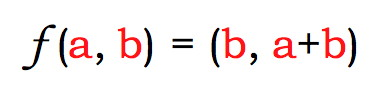☜ Navigate through the Golden Ratio pages ☞

# Fibonacci Pairs

The operation of creating Fibonacci pairs is the same as each step in evaluating the continued fraction of φ.

## Brief Explanation

In the previous page you were introduced to the Fibonacci series. A Fibonacci pair is a pair of adjacent numbers in the series. Their ratio approaches φ. Here we will see why, by looking at the operation that takes a Fibonacci pair and yields the next one.

## Try It Yourself

Here you create Fibonacci pairs. Each time you click the button, you add the two numbers in the pair to find the next value. To start over, click the button.

## Remarks

We can express this operation as a function that takes two numbers and yields two numbers, like this:But now consider the form that each step takes as you evaluate the continued fraction for φ.Your previous exhaustive efforts have got you to this stage. The next step is to add 1 to a/b and then take its reciprocal. But...(You should check the algebra. It will teach you something!)

In other words, the operation you keep repeating is this one:That should look familiar: it is essentially the same as the earlier function labeled with a . It takes two numbers, adds them, and replaces one with the sum. This is the reason that the φ-bonacci numbers (I couldn’t resist the pun) keep appearing in the partial sums of the Golden Ratio.

☜ Navigate through the Golden Ratio pages ☞